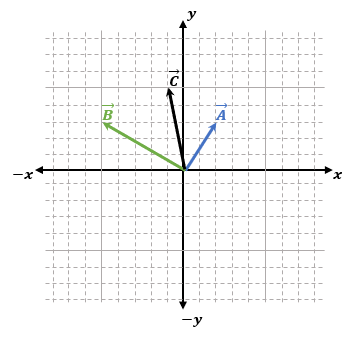## Physics

Learn the toughest concepts covered in Physics with step-by-step video tutorials and practice problems by world-class tutors

3. Vectors

1
concept5m
Play a video:
2
Problem

A delivery truck travels 8 miles in the +x-direction, 5 miles in the +y-direction, and 4 miles again in the +x-direction. What is the magnitude (in miles) of its final displacement from the origin?

3
example2m
Play a video:
Hey, guys, let's work this one out together. We're gonna find the magnitude of a resultant vector by adding up these three vectors over here. So let's check it out. So we've got these three vectors, but they all actually start from the same place, the origin. Which means we're gonna have to connect them tip to tail, that we're gonna find the shortest path from start the first to the end of the last one. That's what always we do for Vector Edition. So let's check it out. So we have these the a vector that's already drawn from the origin. And so all have to do is just connect the B vector tip to tail. So I've got this be vector here. But if you think about this, this be vector goes three to the left and then it goes five up. So the one way I can sort of move it over is start from the end of a and I could just go three the left and then five up. And then my results in our sorry the B vector is just gonna be right here. So basically, I'm kind of like transplanting it over. So that it lines up tip to tail. So this is my B vector. Kind of just erases because I don't need it anymore. Now, my see, vector is gonna be the same thing. It's gonna be five to the left and two down. You're just gonna add it now? Tip to tail from this point over here. So you go. 12345 and then you go. 12 you're gonna end up over here. And so now this is going to be my see Vector added tip to tail. So this is C, which means I don't need it anymore. Notice how all these things are also parallel. So this PC vector is parallel. D. C vectors are parallel. That just means that you drew them in the right way. So that means now that my result in vector is gonna be the start of the first to the end of the last. Actually, instead of you walking this whole path from A to B to C, it is actually it just if you walked in a straight line that connects these two points So this is my d vector here. Now I just have to figure out the magnitude. So I'm just gonna break it down into a triangle, figure out the legs, just point counting up the squares. So I've got this triangle over here and I've got 123456 And then 123456 So I've got a six and six triangle. So now, to calculate the magnitude, you just use the Pythagorean theorem and you have to worry about the signs. Just plug in the numbers of the boxes. Six squared plus six squared and you just get 8.5. And if you wanted, if it was meters or something like that, then you just specify the units. So that's the resultant or that is the magnitude of that. Resultant. Let me know if you guys have any questions.
4
concept

## Subtracting Vectors Graphically5m
Play a video:
5
Problem

Find the magnitude of the Resultant Vector D=C-B-A.6
concept